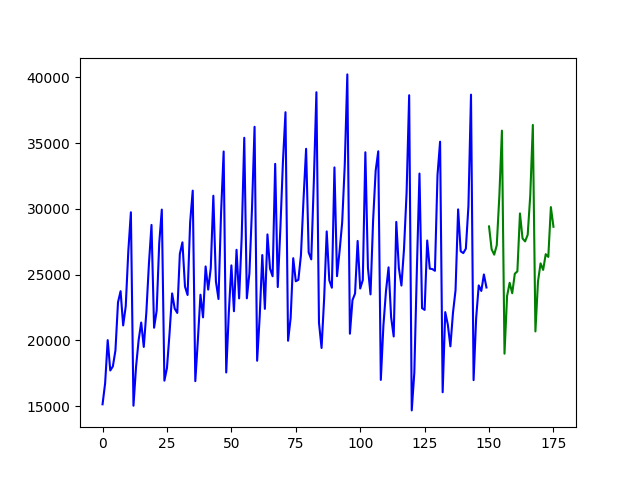# pmdarimaPmdarima (originally `pyramid-arima`, for the anagram of 'py' + 'arima') is a statistical library designed to fill the void in Python's time series analysis capabilities. This includes:

• The equivalent of R's `auto.arima` functionality
• A collection of statistical tests of stationarity and seasonality
• Time series utilities, such as differencing and inverse differencing
• Numerous endogenous and exogenous transformers and featurizers, including Box-Cox and Fourier transformations
• Seasonal time series decompositions
• Cross-validation utilities
• A rich collection of built-in time series datasets for prototyping and examples
• Scikit-learn-esque pipelines to consolidate your estimators and promote productionization

Pmdarima wraps statsmodels under the hood, but is designed with an interface that's familiar to users coming from a scikit-learn background.

## Installation

Pmdarima has binary and source distributions for Windows, Mac and Linux (`manylinux`) on pypi under the package name `pmdarima` and can be downloaded via `pip`:

``````\$ pip install pmdarima
``````

## Quickstart Examples

Fitting a simple auto-ARIMA on the `wineind` dataset:

``````import pmdarima as pm
from pmdarima.model_selection import train_test_split
import numpy as np
import matplotlib.pyplot as plt

train, test = train_test_split(y, train_size=150)

model = pm.auto_arima(train, seasonal=True, m=12)

forecasts = model.predict(test.shape)  # predict N steps into the future

# Visualize the forecasts (blue=train, green=forecasts)
x = np.arange(y.shape)
plt.plot(x[:150], train, c='blue')
plt.plot(x[150:], forecasts, c='green')
plt.show()
``````Fitting a more complex pipeline on the `sunspots` dataset, serializing it, and then loading it from disk to make predictions:

``````import pmdarima as pm
from pmdarima.model_selection import train_test_split
from pmdarima.pipeline import Pipeline
from pmdarima.preprocessing import BoxCoxEndogTransformer
import pickle

train, test = train_test_split(y, train_size=2700)

# Define and fit your pipeline
pipeline = Pipeline([
('boxcox', BoxCoxEndogTransformer(lmbda2=1e-6)),  # lmbda2 avoids negative values
('arima', pm.AutoARIMA(seasonal=True, m=12,
suppress_warnings=True,
trace=True))
])

pipeline.fit(train)

# Serialize your model just like you would in scikit:
with open('model.pkl', 'wb') as pkl:
pickle.dump(pipeline, pkl)

# Load it and make predictions seamlessly:
with open('model.pkl', 'rb') as pkl:
print(mod.predict(15))
# [25.20580375 25.05573898 24.4263037  23.56766793 22.67463049 21.82231043
# 21.04061069 20.33693017 19.70906027 19.1509862  18.6555793  18.21577243
# 17.8250318  17.47750614 17.16803394]
``````

### Availability

`pmdarima` is available on PyPi in pre-built Wheel files for Python 3.6+ for the following platforms:

• Mac (64-bit)
• Linux (64-bit manylinux)
• Windows (32 & 64-bit)

If a wheel doesn't exist for your platform, you can still `pip install` and it will build from the source distribution tarball, however you'll need `cython>=0.29` and `gcc` (Mac/Linux) or `MinGW` (Windows) in order to build the package from source.

Note that legacy versions (<1.0.0) are available under the name "`pyramid-arima`" and can be pip installed via:

``````# Legacy warning:
\$ pip install pyramid-arima
# python -c 'import pyramid;'
``````

However, this is not recommended.

## Documentation

All of your questions and more (including examples and guides) can be answered by the `pmdarima` documentation. If not, always feel free to file an issue.

Get A Weekly Email With Trending Projects For These Topics
No Spam. Unsubscribe easily at any time.
Python (1,124,836
Machine Learning (30,060
Time Series (1,883
Forecasting (457
Econometrics (206
Arima (125
Related Projects